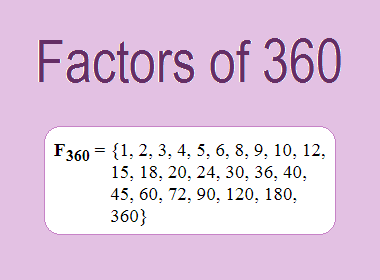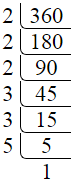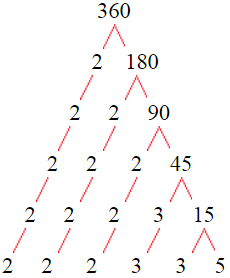# Factors of 360The factors of 360 are 1, 2, 3, 4, 5, 6, 8, 9, 10, 12, 15, 18, 20, 24, 30, 36, 40, 45, 60, 72, 90, 120, 180, and 360 i.e. F360 = {1, 2, 3, 4, 5, 6, 8, 9, 10, 12, 15, 18, 20, 24, 30, 36, 40, 45, 60, 72, 90, 120, 180, 360}. The factors of 360 are all the numbers that can divide 360 without leaving a remainder.

We can check if these numbers are factors of 360 by dividing 360 by each of them. If the result is a whole number, then the number is a factor of 360. Let's do this for each of the numbers listed above:

·        1 is a factor of 360 because 360 divided by 1 is 360.

·        2 is a factor of 360 because 360 divided by 2 is 180.

·        3 is a factor of 360 because 360 divided by 3 is 120.

·        4 is a factor of 360 because 360 divided by 4 is 90.

·        5 is a factor of 360 because 360 divided by 5 is 72.

·        6 is a factor of 360 because 360 divided by 6 is 60.

·        8 is a factor of 360 because 360 divided by 8 is 45.

·        9 is a factor of 360 because 360 divided by 9 is 40.

·        10 is a factor of 360 because 360 divided by 10 is 36.

·        12 is a factor of 360 because 360 divided by 12 is 30.

·        15 is a factor of 360 because 360 divided by 15 is 24.

·        18 is a factor of 360 because 360 divided by 18 is 20.

·        20 is a factor of 360 because 360 divided by 20 is 18.

·        24 is a factor of 360 because 360 divided by 24 is 15.

·        30 is a factor of 360 because 360 divided by 30 is 12.

·        36 is a factor of 360 because 360 divided by 36 is 10.

·        40 is a factor of 360 because 360 divided by 40 is 9.

·        45 is a factor of 360 because 360 divided by 45 is 8.

·        60 is a factor of 360 because 360 divided by 60 is 6.

·        72 is a factor of 360 because 360 divided by 72 is 5.

·        90 is a factor of 360 because 360 divided by 90 is 4.

·        120 is a factor of 360 because 360 divided by 120 is 3.

·        180 is a factor of 360 because 360 divided by 180 is 2.

·        360 is a factor of 360 because 360 divided by 360 is 1.

## How to Find Factors of 360?

1 and the number itself are the factors of every number. So, 1 and 360 are two factors of 360. To find the other factors of 360, we can start by dividing 360 by the numbers between 1 and 360. If we divide 360 by 2, we get a remainder of 0. Therefore, 2 is a factor of 360. If we divide 360 by 3, we get a remainder of 0. Therefore, 3 is also a factor of 360.

Next, we can check if 4 is a factor of 360. If we divide 360 by 4, we get a remainder of 0. Therefore, 4 is also a factor of 360. We can continue this process for all the possible factors of 360.

Through this process, we can find that the factors of 360 are 1, 2, 3, 4, 5, 6, 8, 9, 10, 12, 15, 18, 20, 24, 30, 36, 40, 45, 60, 72, 90, 120, 180, and 360. These are the only numbers that can divide 360 without leaving a remainder.

********************

********************

## Properties of the Factors of 360

The factors of 360 have some interesting properties. One of the properties is that the sum of the factors of 360 is equal to 1170. We can see this by adding all the factors of 360 together:

1 + 2 + 3 + 4 + 5 + 6 + 8 + 9 + 10 + 12 + 15 + 18 + 20 + 24 + 30 + 36 + 40 + 45 + 60 + 72 + 90 + 120 + 180 + 360 = 1170

Another property of the factors of 360 is that the prime factors of 360 are 2, 3, and 5 only.

## Applications of the Factors of 360

The factors of 360 have several applications in mathematics. One of the applications is in finding the highest common factor (HCF) of two or more numbers. The HCF is the largest factor that two or more numbers have in common. For example, to find the HCF of 360 and 84, we need to find the factors of both numbers and identify the largest factor they have in common. The factors of 360 are 1, 2, 3, 4, 5, 6, 8, 9, 10, 12, 15, 18, 20, 24, 30, 36, 40, 45, 60, 72, 90, 120, 180, and 360. The factors of 84 are 1, 2, 3, 4, 6, 7, 12, 14, 21, 28, 42, and 84. The largest factor that they have in common is 12. Therefore, the HCF of 360 and 84 is 12.

Another application of the factors of 360 is in prime factorization. Prime factorization is the process of expressing a number as the product of its prime factors. The prime factors of 360 are 2, 3, and 5 since these are the only prime numbers that can divide 360 without leaving a remainder. Therefore, we can express 360 as:

360 = 2 × 2 × 2 × 3 × 3 × 5

We can do prime factorization by division and factor tree method also. Here is the prime factorization of 360 by division method,360 = 2 × 2 × 2 × 3 × 3 × 5

Here is the prime factorization of 360 by the factor tree method,360 = 2 × 2 × 2 × 3 × 3 × 5

## Conclusion

The factors of 360 are the numbers that can divide 360 without leaving a remainder. The factors of 360 are 1, 2, 3, 4, 5, 6, 8, 9, 10, 12, 15, 18, 20, 24, 30, 36, 40, 45, 60, 72, 90, 120, 180, and 360. The factors of 360 have some interesting properties, such as having a sum of 1170. The factors of 360 have several applications in mathematics, such as finding the highest common factor and prime factorization.